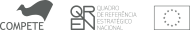Bôcher, Maxime
An introduction to the study of integral equations / Maxime Bôcher . - New York : Hafner, [1950 - 1960?] . - 70 p . - (Cambridge tracts in mathematics and mathematical physics ; 10)

Ed. orig.: 1913?.

CDU 51© 2017 Universidade de Aveiro